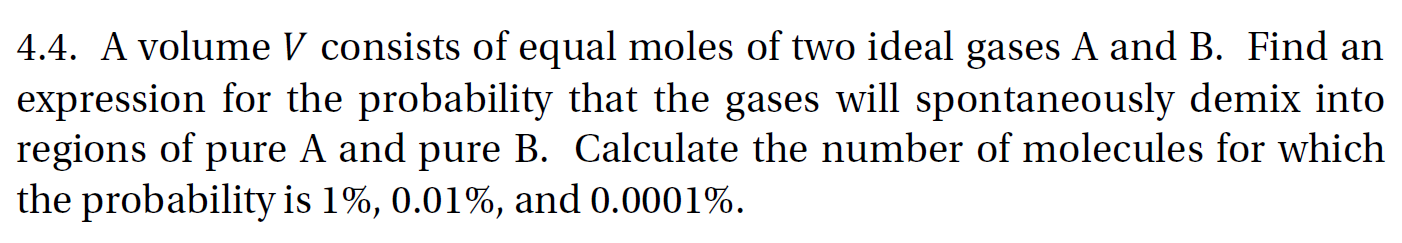# 4.4. A volume V consists of equal moles of two ideal gases A and B. Find anexpression for the probability that the gases will spontaneously demix intoregions of pure A and pure B. Calculate the number of molecules for whichthe probability is 1%, 0.01%, and 0.0001%.

Question

question 4.4  from thermodynamics and statistical mechanics an integrated approach written by scott shell

A volume V consists of equal moles of two ideal gases A and B. Find an
expression for the probability that the gases will spontaneously demix into
regions of pure A and pure B. Calculate the number of molecules for which
the probability is 1%, 0.01%, and 0.0001%.help_outlineImage Transcriptionclose4.4. A volume V consists of equal moles of two ideal gases A and B. Find an expression for the probability that the gases will spontaneously demix into regions of pure A and pure B. Calculate the number of molecules for which the probability is 1%, 0.01%, and 0.0001%. fullscreen
check_circle

Step 1

The expression for the probability is,

Step 2

The number of molecules when probability 1%,

Step 3

The number of molecules whe...

### Want to see the full answer?

See Solution

#### Want to see this answer and more?

Solutions are written by subject experts who are available 24/7. Questions are typically answered within 1 hour.*

See Solution
*Response times may vary by subject and question.
Tagged in

### Physics